# Visualization with Seaborn – Part 1 – Distribution and Categorical PlotsShare this article!

## Visualizing Your Data With Seaborn.

Seaborn is an extremely well-built library for Data Visualization. It can build beautiful plots to efficiently visualize your data.
Have a look at the official documentation here, and see the various kinds of plots that we can make using Seaborn.

In this tutorial, we will look at some of the most important plot types.

Let’s start off by importing the package:

In :
```import seaborn as sns
%matplotlib inline
```

For the Data, we will use one of the included data sets of Seaborn. Yes, seaborn actually comes with some built-in data sets!

In :
```tips = sns.load_dataset('tips')
```

Lets’s check the head of our data as always.

In :
```tips.head()
```
Out:
total_bill tip sex smoker day time size
0 16.99 1.01 Female No Sun Dinner 2
1 10.34 1.66 Male No Sun Dinner 3
2 21.01 3.50 Male No Sun Dinner 3
3 23.68 3.31 Male No Sun Dinner 2
4 24.59 3.61 Female No Sun Dinner 4

So this Data set is about the people who visited a restaurant and left a tip. It has seven columns:

1. total_bill: Total bill of the table
2. tip: The tip amount left
3. sex: Customer’s gender
4. smoker: Weather or not the customer is a smoker
5. day: the particular day of the week
6. time: either lunch or dinner
7. size: The number of members in the group

Let’s move to the visualization part.

First of all, we will have a look at Distribution Plots.

## Distribution Plots

They essentially allow us to visualize the distribution of the data. There are a few kinds of distribution plots that we are going to see.

### distplot

The distplot shows the distribution of any one variable of the data set. Let’s go ahead and see the distribution of “total_bill”.

In :
```sns.distplot(tips['total_bill'])
```
Out:
`<matplotlib.axes._subplots.AxesSubplot at 0xbe7c2e8>`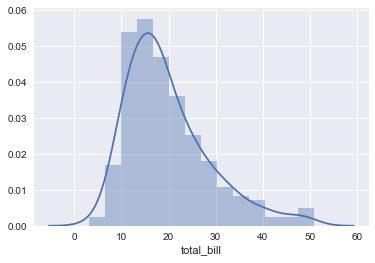This is essentially an Histogram. The line we see is called “kde layer”. We will talk about it in some time. For now,you can remove it by using kde=False argument. Also, we can change the number of bins by using bins argument.

In :
```sns.distplot(tips['total_bill'],kde=False,bins=30)
```
Out:
`<matplotlib.axes._subplots.AxesSubplot at 0xbf7e518>`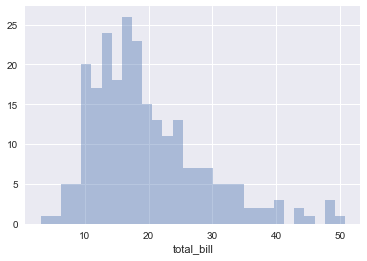A histogram shows where most of your distribution lies. Here, we can see that most of the values of total_bill lie somewhere between 15 and 20. Play around with this plot using different variables and number of bins.

Next up is:

### jointplot

It basically combines two distplots. We can therefore have two variables.
In this, we pass in an “x” variable, a “y” variable, the “data”, and “kind” of plot.
the kind can be any of the following:

• “scatter”
• “reg”
• “resid”
• “kde”
• “hex”

Let’s see the distribution of total_bill and corresponding tips with scatter plot.

In :
```sns.jointplot(x='total_bill',y='tip',data=tips,kind='scatter')
```
Out:
`<seaborn.axisgrid.JointGrid at 0xc263cf8>`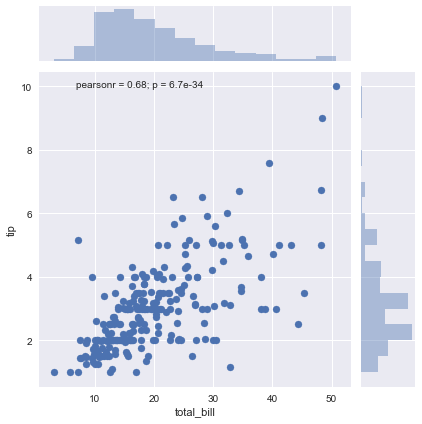So we see here two distplots: tip on the y-axis, and total_bill on the x-axis, and a scatter plot between them. Go ahead and try the other “kind” attributes.

Let’s explore the next kind of plot:

### pairplot

Pairplot will plot a joint plot for every possible combination of the numerical columns in the whole dataframe. we just need to pass the complete data.

In :
```sns.pairplot(tips)
```
Out:
`<seaborn.axisgrid.PairGrid at 0xc935be0>`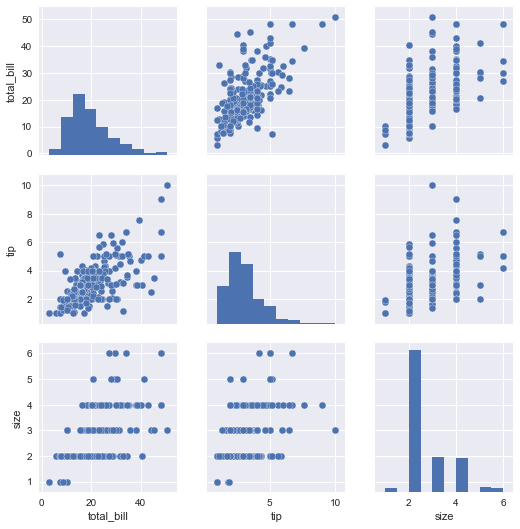You can see scatter plots for every combination of numerical columns, except for same columns in which case a scatter plot won’t make sense. This helps to quickly visualize the data. The cool thing about it is the hue parameter that we can pass to visualize the categorical columns as well.

In :
```sns.pairplot(tips,hue='sex',palette='husl')
```
Out:
`<seaborn.axisgrid.PairGrid at 0x1061e240>`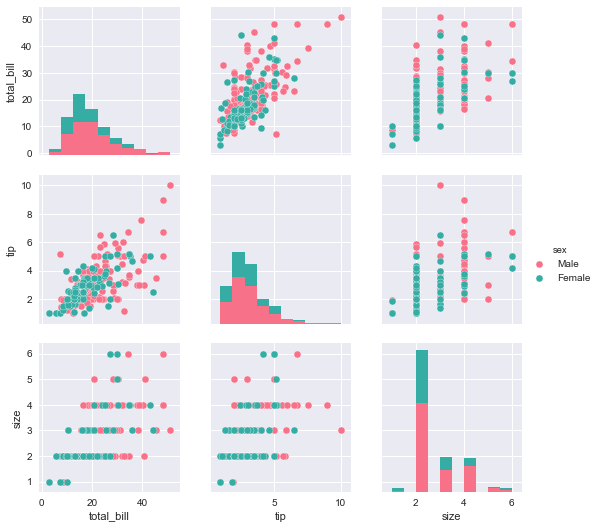Now, the “male” and “female” data is colored differently. An easy-peasy way of determining clusters! Play around with the palette attribute which defines the color scheme.

Let’s move on to the next type of plots.

## Categorical Plots

Now we will plot the categorical variables such as sex, smoker, day, and time.
The most basic type is the Bar Plot.

### barplot

These essentially plot the aggregated data for the desired category. Let’s see a simple example.

In :
```sns.barplot(x='sex',y='total_bill',data=tips)
```
Out:
`<matplotlib.axes._subplots.AxesSubplot at 0x116ca1d0>`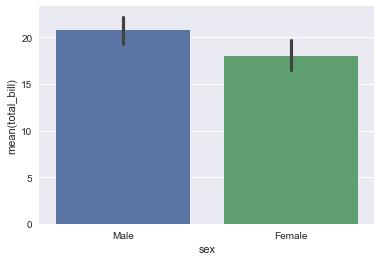By default, the aggregate function used is “mean”. So this plot is just showing the mean values of total_bill for make and female guests. The aggregate function can be changed by using the estimator argument, but more on that later.

A very similar one is the next plot that we are going to discuss.

### countplot

It is basically same as the the barplot, except that the aggregate function it uses is the total count of values of each category. Hence it only requires the x variable.

In :
```sns.countplot(x='sex',data=tips)
```
Out:
`<matplotlib.axes._subplots.AxesSubplot at 0x112e2828>`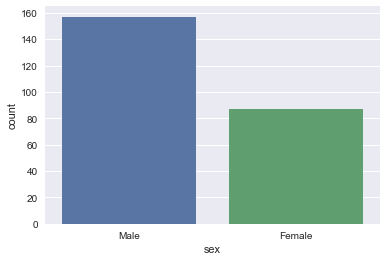Let’s get to some more informative plots.

### boxplot

These are used to show the distribution of the categorical variables. Let’s examine an example.

In :
```sns.boxplot(x="day", y="total_bill", data=tips)
```
Out:
`<matplotlib.axes._subplots.AxesSubplot at 0x118c4668>`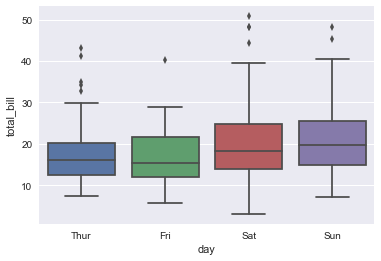So we have plotted total_bill corresponding to each day. The boxes in the figure show the “quartiles” of the data. The few dots on the top are interpreted as the outliers.

We can add “hue” and “palette” attributes to this plot as well.

In :
```sns.boxplot(x="day", y="total_bill",data=tips, hue="smoker", palette="rainbow")
```
Out:
`<matplotlib.axes._subplots.AxesSubplot at 0x1214d6a0>`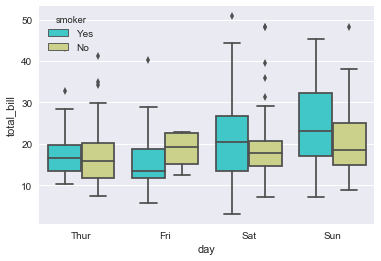Now, for each day, there are two box plots:
one corresponding to to smokers,
and the other to non-smokers.

You can see that in general, smokers pay more bill than non-smokers, except on Fridays.

Let’s move to an advanced plot for categorical data.

### stripplot

Strip plot is used to draw a scatter plot for the categorical data.

In :
```sns.stripplot(x="day", y="total_bill", data=tips)
```
Out:
`<matplotlib.axes._subplots.AxesSubplot at 0x125251d0>`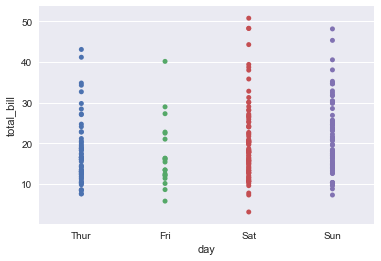As we can see, the scatter dots are overlapping, making it difficult to estimate the density. We can use the jitter parameter to solve this problem.

In :
```sns.stripplot(x="day", y="total_bill", data=tips,jitter=True)
```
Out:
`<matplotlib.axes._subplots.AxesSubplot at 0x1251cc50>`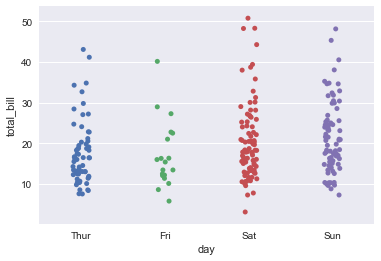Now it’s much easier to analyse the density. As others, here also we can add the “hue” and “palette” parameters.

In :
```sns.stripplot(x="day", y="total_bill", data=tips,jitter=True,hue='sex',palette='Set1')
```
Out:
`<matplotlib.axes._subplots.AxesSubplot at 0x126fc358>`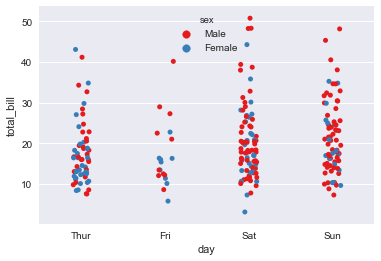Quite a few plots to visualize your data as and how you want!

But, that’s not all. Seaborn has many more useful plots in store for you.
These will be discussed in the next part of Visualization with Seaborn series. Stay updated.

Comment for any doubt. Happy learning 🙂

Share this article!### 2 Comments

1.Debayan

Even better than Seaborn is plotly …it creates very detailed interactive plots which are very beautiful to look at and also the dashboard created by the same are very interactive .

•Tanishk Sachdeva

Hey Debayan, indeed plotly and cufflinks can be used to create plots that are interactive and pleasing to share information. We have just given seaborn an edge to plot simple data, though matplotlib remains at the top when it comes to customization.